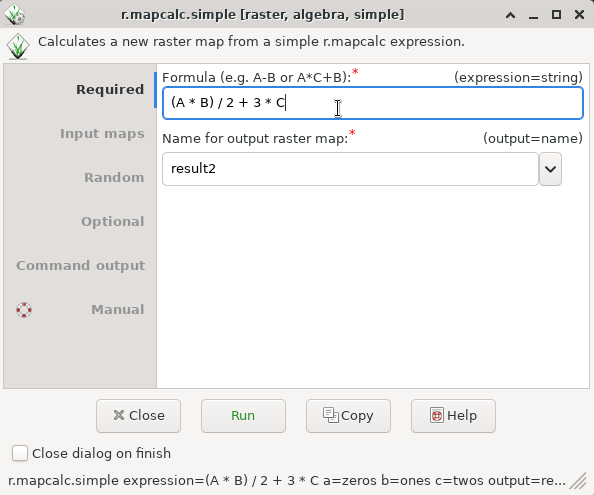Note: This document is for an older version of GRASS GIS that will be discontinued soon. You should upgrade, and read the current manual page.

## NAME

r.mapcalc.simple - Calculates a new raster map from a simple r.mapcalc expression.

## KEYWORDS

raster, algebra, simple

## SYNOPSIS

r.mapcalc.simple
r.mapcalc.simple --help
r.mapcalc.simple [-sqc] expression=string [a=name] [b=name] [c=name] [d=name] [e=name] [f=name] output=name [seed=integer] [--overwrite] [--help] [--verbose] [--quiet] [--ui]

### Flags:

-s
Generate random seed (result is non-deterministic)
-q
Quote the map names
-c
Case sensitive variable names
--overwrite
Allow output files to overwrite existing files
--help
Print usage summary
--verbose
Verbose module output
--quiet
Quiet module output
--ui
Force launching GUI dialog

### Parameters:

expression=string [required]
Formula (e.g. A-B or A*C+B)
a=name
Name of input A raster map
b=name
Name of input B raster map
c=name
Name of input C raster map
d=name
Name of input D raster map
e=name
Name of input E raster map
f=name
Name of input F raster map
output=name [required]
Name for output raster map
seed=integer
Seed for rand() function

## DESCRIPTION

r.mapcalc.simple provides a wrapper to r.mapcalc. Up to 6 maps can be combined using simple expressions.

The general syntax for the expression follows r.mapcalc expression format, for example, A + B or exp(A + B) are valid. The variables A, B, ..., F represent raster maps which are provided as options a, b, ..., f.

The result name, i.e. the output raster map, is provided using the option output and, unlike r.mapcalc it is not part of the expression.

This module is meant for convenience (for users and programmers) while the r.mapcalc module is a better choice for more complex expressions and advanced usage.

## NOTES

Differences to r.mapcalc module:
• The input raster map names and the output map raster name are separate from the expression (formula) which uses generic variable names (A, B, C, ...).
• The output raster name is not included in the expression.
• The expression is expected to be a single short one liner without the function eval().
Differences to r.mapcalc.simple module in GRASS GIS 5 and 6:
• The primary purpose is not being a GUI front end to r.mapcalc, but a wrapper which allows easy building of interfaces to r.mapcalc (including GUIs).
• Whitespace (most notably spaces) are allowed (in the same way as for r.mapcalc).
• The variable names are case-insensitive to allow the original uppercase as well as lowercase as in option names (unless the -c flag is used).
• Option names for each map are just one letter (not amap, etc.).
• Output option name is output as for other modules (not outfile).
• Raster map names can be optionally quoted (the -q flag).
• There is no expert mode (which was just running r.mapcalc).
• The expression option is first, so it is possible to omit its name in the command line (just like with r.mapcalc).
• Overwriting of outputs is done in the same way as with other modules, so there is no flag to not overwrite outputs.

## EXAMPLES

### Basic examples

```r.mapcalc.simple expression="0" output=zeros
r.mapcalc.simple expression="1" output=ones
r.mapcalc.simple expression="2" output=twos
```
```r.mapcalc.simple expression="A + B + C" a=zeros b=ones c=twos output=result1
```
```r.mapcalc.simple expression="(A * B) / 2 + 3 * C" a=zeros b=ones c=twos output=result2
```Figure: r.mapcalc.simple graphical user interface

### Example expressions

```A + B
```
No spaces around operators are not recommended for readability, but allowed in the expression:
```A+B
```
More complex expression with a function:

```exp(A+C)+(B-2)*7
```

r.mapcalc, r3.mapcalc, t.rast.mapcalc, g.region

## AUTHORS

Vaclav Petras, NCSU GeoForAll Lab
Michael Barton, Arizona State University (updated to GRASS 5.7)
R. Brunzema (original 5.0 Bash version)

## SOURCE CODE

Available at: r.mapcalc.simple source code (history)

Latest change: Thursday Feb 03 11:10:06 2022 in commit: 547ff44e6aecfb4c9cbf6a4717fc14e521bec0be

Note: This document is for an older version of GRASS GIS that will be discontinued soon. You should upgrade, and read the current manual page.

© 2003-2023 GRASS Development Team, GRASS GIS 8.2.2dev Reference Manual